# Catch up on the latest AI articles# Small Neural Networks Should Augmentation The Network!

3 main points
✔️ Proposed NetAug (Network Augmentation) to improve the accuracy of small neural networks
✔️ Extend the network to mitigate underfitting
✔️ Consistent performance improvement in image classification and object detection tasks

written by Han CaiChuang GanJi LinSong Han
(Submitted on 17 Oct 2021 (v1), last revised 24 Apr 2022 (this version, v2))

Subjects: Computer Vision and Pattern Recognition (cs.CV); Artificial Intelligence (cs.AI); Machine Learning (cs.LG)code：The images used in this article are from the paper, the introductory slides, or were created based on them.

## first of all

Various regularization techniques (e.g., data augmentation and dropout) have been used with much success to improve the performance of deep learning.

However, these existing regularization methods may rather impair the performance of small neural networks, where overfitting is less likely to occur. In the paper presented in this article, we proposed NetAug, a method to augment the network rather than the data, as a method to improve the performance of small neural networks.

The results include an accuracy improvement of up to 2.2% on ImageNet for small-scale models.

## NetAug(Network Augmentation)

### Formulation of Network Augmentation

First, let $W_t$ be the weights of the small (tiny) neural network that we want to train and $L$ be the loss function. During training, the weights are optimized to minimize $L$.

$W^{n+1}_t = W^n_t - \eta \frac{\partial L(W^n_t)}{\partial W^n_t}$

At this time, small neural networks tend to fall into locally optimal solutions and have poorer training and testing performance than large neural networks due to their small capacity.

The proposed method, Network Augmentation (Network Augmentation), introduces additional supervision by a larger neural network to assist in the training of a smaller neural network.

This can be said to be the opposite of the dropout method, which makes predictions only on a part of the network, and creates a huge neural network by extending the width of the small neural network you want to train.

The idea can be summarized in the following diagram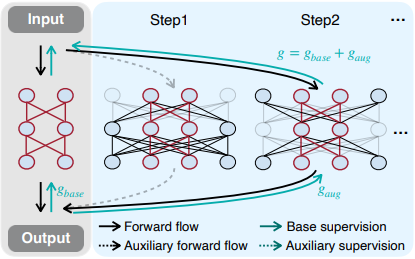The loss function $L_{aug}$ in the network augmentation then becomes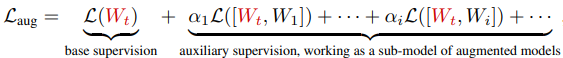where $[W_t, W_i]$ represents the small neural network $W_t$ that we want to train and the extended model including new weights $W_i$.

$\alpha_i$ is a hyperparameter to integrate the losses of different augmented models.

When augmenting a network, it is difficult to make the augmented model $[W_t, W_i]$ independent from the aspect of computational resources. Therefore, we construct various augmented models in the form of selecting the subnetwork of the largest model (see Step1 and Step2 in the aforementioned figure).

The idea is similar to One-Shot NAS, except that the focus is on improving the performance of a particular small neural network, rather than on obtaining the optimal subnetwork.

In addition, when the network is augmented, the augmentation is done in such a way that the width of the model is increased.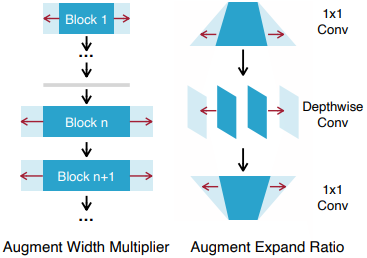### learning process

When training the augmented network, the model is updated by sampling the subnetworks at each step.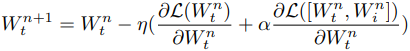Where $[W^n_t, W^n_i]$ is the network sampled at each learning step. The $W_i$ of the augmented part is updated as well as the weights of the small neural network we want to learn.

Note that the hyperparameter $\alpha$ is fixed at 1.0 for all experiments.

### Overheads in Learning and Inference

First, network augmentation is done only at training time, so there is no overhead at inference time. For learning time, our experiments show that the learning time is only 16.7% longer.

This is because network augmentation is assumed to be used for training small networks, and the data loading and communication costs dominate over the computational processing during training.

## experimental setup

The image classification dataset and training settings used in the experiments are as follows.

• ImageNet: 16 GPUs, 150 epochs with batch size 2048
• ImageNet-21K-P(winter21 version):16GPU, 20 epochs with batch size 2048
• Food101: initialized with pre-trained weights in ImageNet, 50 epochs of fine-tuning on 4 GPUs with batch size 256
• Flowers102:Same as Food101
• Similar to Cars: Food101
• Cub200: Same as Food101
• Similar to Pets: Food101

The image detection dataset is also shown below.

• Pascal VOC: 8 GPUs, batch size 64 for 200 epochs
• COCO: 16 GPUs, 120 epochs with batch size 128

## experimental results

First, the results in ImageNet are as follows.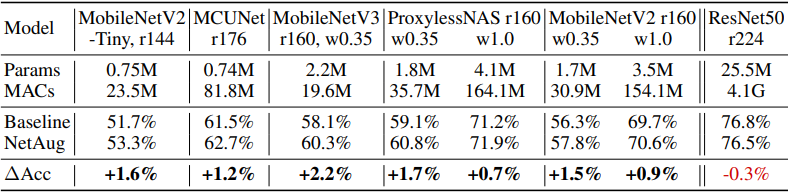In general, the proposed method can consistently improve the accuracy of small neural networks for different neural network architectures.

Note that network augmentation is a method that is supposed to be applied to small neural networks, so ResNet50 with sufficient model capacity will not improve the accuracy. The plot of accuracy against the number of training epochs is shown below.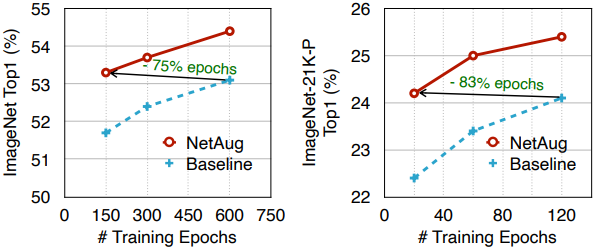As shown in the figure, the number of training epochs required to achieve the same accuracy is significantly reduced compared to the no-enhancement case.

### Comparison with Knowledge Distillation

Next, the comparison with knowledge distillation using the Assemble-ResNet50 is a supervised model is as follows.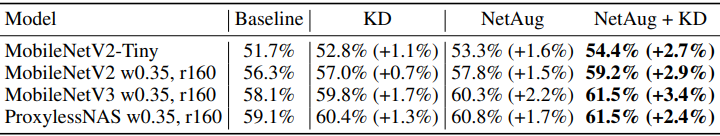In general, a high improvement in accuracy is achieved compared to the knowledge distillation method. It is also found that the proposed method can be combined with knowledge distillation, in which case the accuracy is further improved significantly.

### Comparison with regularization methods

Next, the comparison results with dropout, data augmentation, and other methods that lead to improved accuracy for large neural networks are shown below.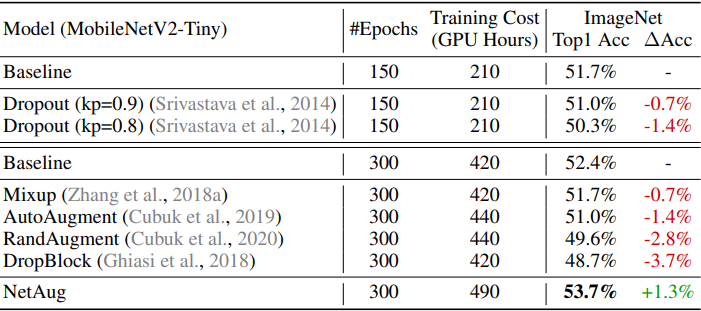In general, small neural networks suffer more from underfitting than overfitting, so methods to prevent overfitting are counterproductive.

Conversely, the proposed method of network augmentation is designed to mitigate the underfitting of small neural networks, so applying it to large neural networks is counterproductive. These are shown in the following learning curves.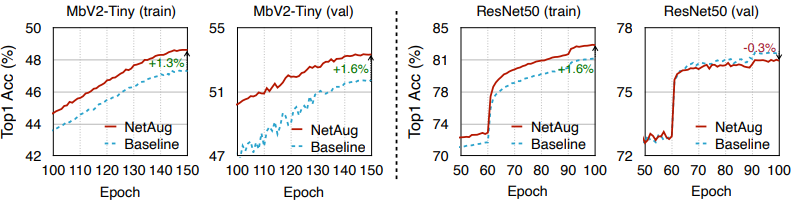In the figure, the train/val accuracy plots for a small neural network (MobileNetV2-Tiny) and a large neural network (ResNet50) are shown.

As mentioned earlier, both train/val accuracy has improved for the small neural network, while the val accuracy has decreased for ResNet, showing signs of overfitting.

### Results in transfer learning

Finally, the following results are obtained when we perform the transition learning of the model pre-trained by ImageNet.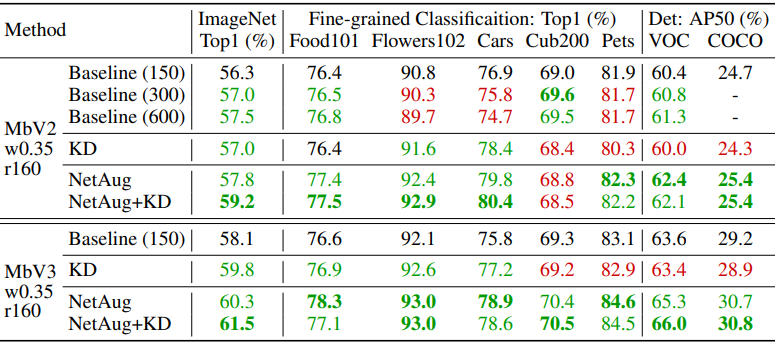Compared to the baseline (Baseline(150)), better results are shown in green and worse results in red. Overall, we found that Network Augmentation performed well when learning transitions.

The proposed method also performs well at smaller resolutions, which improves inference efficiency. The following figures show the results of object detection at different resolutions.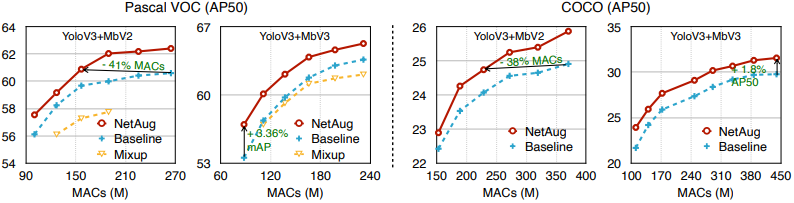In this figure, we can see that we can achieve comparable performance at smaller resolutions.

## summary

In this article, we introduced Network Augmentation, a method for improving the performance of small neural networks that are prone to suffer from underfitting. The method was shown to consistently improve accuracy in tasks such as image classification and object detection.

Existing regularization methods are mainly aimed at dealing with the overfitting of large neural networks, but given that we want to use small models for edge devices, etc., it is also important to use such small neural network-specific improvement methods.

If you have any suggestions for improvement of the content of the article,